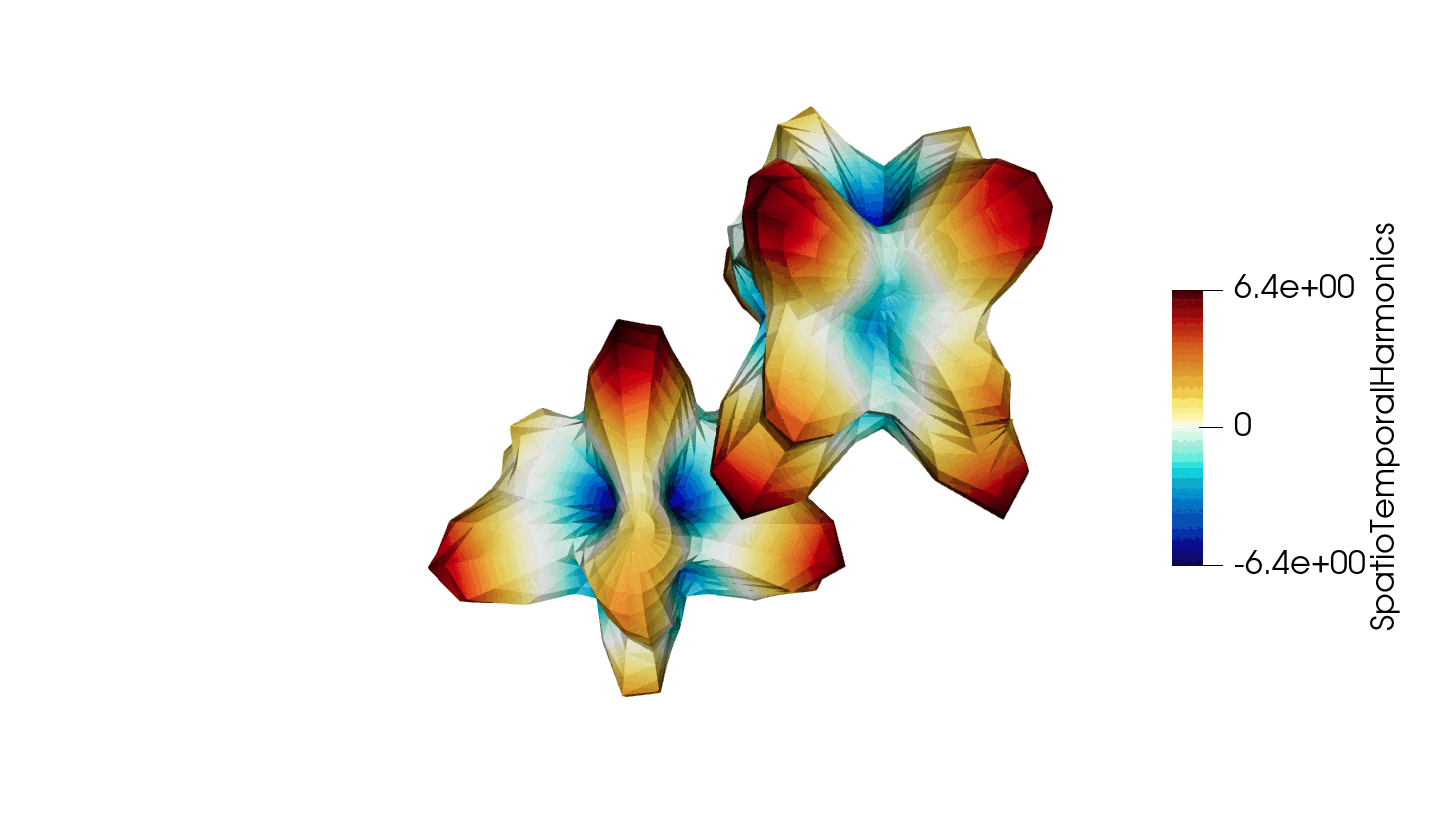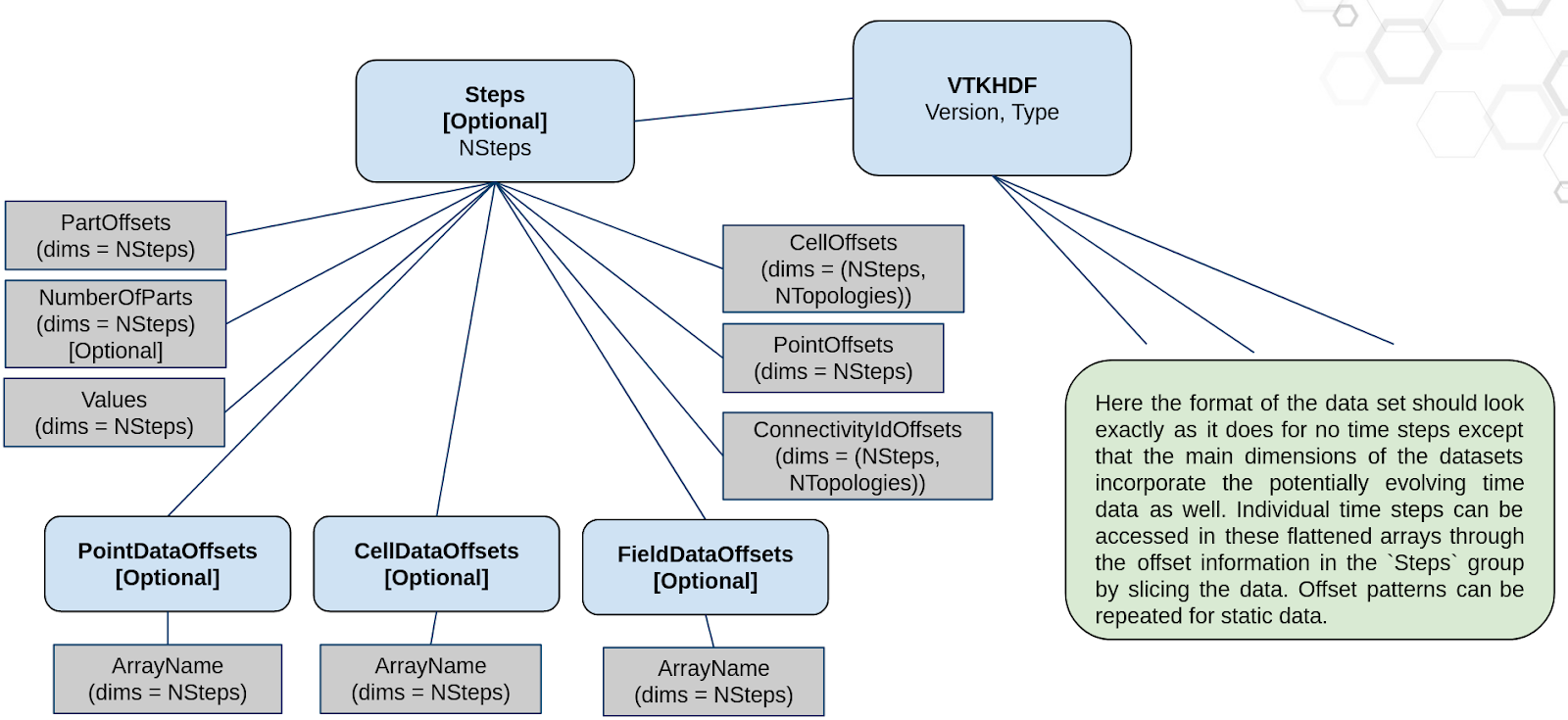# How to write time dependent data in VTKHDF files

October 27, 2023Thanks to recent developments, the VTKHDF format now supports storing time dependent data in a single HDF5 file. The details of the specification can be found here: https://docs.vtk.org/en/latest/design_documents/VTKFileFormats.html#transient-data, but for convenience, the synthetic diagram is reproduced below in Figure 1.Figure 1: Schematic diagram of the time dependent meta-data in the VTKHDF format

The idea is that the format looks very much like the non-transient case but with time dependent data flattened and in-lined into the HDF5 “dataset” entries. The supplemental (and optional) “Steps” group then describes the offsets with which to read every time step in the underlying data. This lends flexibility to the format which can then store meshes or data that is static in time by simply manipulating the offset with which it is read in the dataset. The idea here is to have a minimal storage footprint and a maximal feature set with competitive performance.

The goal of this blog post is to serve as a step by step guide on how to write this kind of file using python scripting, `vtkpython` data structures and the `h5py` interface to the HDF5 library (https://pypi.org/project/h5py/). We will explain how to write data to HDF5 files using a `Poly Data` type test case since it has multiple topologies associated with it and the most complete feature coverage. Other types of data structures can be written similarly. As a disclaimer, in the code snippets proposed below, we are not looking for I/O performance but to show how to use the format specifications and its features to write files.

## The test case

Our test case will consist of two spheres that are being warped under a periodic field. As such, it will consist of data that has a moving geometry but a fixed topology and fields that vary in time. This should illustrate the capability of the format to handle both fixed and transient data seamlessly.

The pipeline used to generate this data is schematically described in Figure 2.

Figure 2: Diagram of the data generation pipeline

and the reproduced pipeline in vtkpython:

``````import numpy as np
from vtkmodules.vtkCommonDataModel import vtkDataObject
from vtkmodules.vtkFiltersGeneral import (vtkGroupDataSetsFilter,
vtkSpatioTemporalHarmonicsAttribute,
vtkWarpScalar)
from vtkmodules.vtkFiltersHybrid import vtkGenerateTimeSteps

sphere0 = vtkSphereSource()
sphere0.SetPhiResolution(30)
sphere0.SetThetaResolution(30)

sphere1 = vtkSphereSource()
sphere1.SetPhiResolution(30)
sphere1.SetThetaResolution(30)
sphere1.SetCenter(15, 15, 15)

# store the spheres in a single partitioned data set
groupDataSets = vtkGroupDataSetsFilter()
groupDataSets.SetOutputTypeToPartitionedDataSet()

# generate time steps
timeSteps = vtkGenerateTimeSteps()
timeSteps.SetInputConnection(groupDataSets.GetOutputPort())
timeValues = np.linspace(0.0, 2*np.pi, 100, endpoint=False)
timeSteps.SetTimeStepValues(100, timeValues)

# generate fields
harmonics = np.array([
[1.0, 1.0, 0.6283, 0.6283, 0.6283, 0.0],
[3.0, 1.0, 0.6283, 0.0, 0.0, 1.5708],
[2.0, 2.0, 0.0, 0.6283, 0.0, 3.1416],
[1.0, 3.0, 0.0, 0.0, 0.6283, 4.7124]
])
for iH in range(harmonics.shape):
harmonics[iH, 1],
harmonics[iH, 2],
harmonics[iH, 3],
harmonics[iH, 4],
harmonics[iH, 5])

# warp spheres
warp = vtkWarpScalar()
warp.SetInputArrayToProcess(0, 0, 0,
vtkDataObject.FIELD_ASSOCIATION_POINTS,
'SpatioTemporalHarmonics')

# update the entire thing
warp.Update()
``````Figure 3: Animated illustration of the warped sphere test case

## Setting up the file

Figure 4: Schematic diagram of the Poly Data specification in the VTKHDF format

This section is dedicated to setting up the structure of the file for easily writing to it in subsequent sections. We will be using h5py for its ease of use and widespread adoption in the python community.

The first thing to do is to create the file:

``````import h5py as h5
file = h5.File('warping_spheres.vtkhdf', 'w')``````

Subsequently, we can generate the top level group (or root) and populate its attributes:

``````root = file.create_group('VTKHDF')
root.attrs['Version'] = (2, 0)
typeAsASCII = 'PolyData'.encode('ascii')
root.attrs.create('Type', typeAsASCII,
dtype=h5.string_dtype('ascii', len(typeAsASCII)))``````

At the time of writing the most up to date VTKHDF version is 2.0 and the “Type” attribute should be written as an ASCII string in the file.

We can now create the general structure that will accommodate the geometry data from the warping spheres:

``````root.create_dataset('NumberOfPoints', (0,), maxshape=(None,), dtype='i8')
root.create_dataset('Points', (0, 3), maxshape=(None,3), dtype='f')``````

Indeed, the `maxshape = (None,...)` parameter allows us to indicate that the datasets will be resizable and enable chunking in the h5py framework and that we will be appending to these later in the program.

Creating the topology structures in the file is done similarly:

``````connectivitySets = ['Vertices', 'Lines', 'Polygons', 'Strips']
for connectivitySet in connectivitySets:
connectivityGroup = root.create_group(connectivitySet)
connectivityGroup.create_dataset('NumberOfConnectivityIds',
(0,), maxshape=(None,), dtype='i8')
connectivityGroup.create_dataset('NumberOfCells',
(0,), maxshape=(None,), dtype='i8')
connectivityGroup.create_dataset('Offsets',
(0,), maxshape=(None,), dtype='i8')
connectivityGroup.create_dataset('Connectivity',
(0,), maxshape=(None,), dtype='i8')``````

To accommodate the warping field situated on the nodes of the mesh we can create the `PointData` group and associated dataset:

``````pointData = root.create_group('PointData')
pointData.create_dataset('SpatioTemporalHarmonics',
(0,), maxshape=(None,), dtype='f')``````

Last, but not least, we have the `Steps` group to hold our time dependent meta-data:

``````steps = root.create_group('Steps')
steps.attrs['NSteps'] = 0``````

Then we have the `Values` data set to hold the values of each time step:

``steps.create_dataset('Values', (0,), maxshape=(None,), dtype='f')``

The part offsetting dataset:

``````steps.create_dataset('PartOffsets', (0,), maxshape=(None,), dtype='i8')
steps.create_dataset('NumberOfParts', (0,), maxshape=(None,), dtype='i8')``````

which will tell the reader which part to go to start reading the given time step and how many parts are included in that time step.

We need to create the geometry offsetting structures holding the information of where to start reading point coordinates:

``steps.create_dataset('PointOffsets', (0,), maxshape=(None,), dtype='i8')``

and the equivalent topological offsetting structures:

``````steps.create_dataset('CellOffsets', (0,4), maxshape=(None,4), dtype='i8')
steps.create_dataset('ConnectivityIdOffsets',
(0,4), maxshape=(None,4), dtype='i8')``````

where the `4` value in the second dimension represents the 4 topologies present in Poly Data in order: Vertices, Lines, Polygons and Strips.

Finally, to be able to determine the offsets related to our field defined on the points, we have the point data offsetting structures:

``````pointDataOffsets = steps.create_group('PointDataOffsets')
pointDataOffsets.create_dataset('SpatioTemporalHarmonics',
(0,), maxshape=(None,), dtype='i8')``````

The file is now completely structured and ready to accept our temporal data.

## Writing time steps to the file incrementally

Given that scientific data is often generated incrementally in a time stepping schema, it is important that a file format is capable of storing data incrementally. This is the case for VTKHDF and what follows is dedicated to showing exactly how.

Our first step is to write the static data, that does not change over time, directly into the file. In our warping spheres test case, the connectivity information actually does not change in time.

As such, we can extract the spheres and its connectivities:

``````from vtkmodules.vtkCommonDataModel import (vtkPartitionedDataSet,
vtkPolyData)
output = vtkPartitionedDataSet.SafeDownCast(warp.GetOutputDataObject(0))
parts = []
parts.append(vtkPolyData.SafeDownCast(output.GetPartition(0)))
parts.append(vtkPolyData.SafeDownCast(output.GetPartition(1)))``````

and subsequently load them into the file:

``````def append_dataset(dset, array):
originalLength = dset.shape
dset.resize(originalLength + array.shape, axis=0)
dset[originalLength:] = array

# write the connectivity information of the spheres
from vtkmodules.util.numpy_support import vtk_to_numpy
for part in parts:
cellArrays = [part.GetVerts(),
part.GetLines(),
part.GetPolys(),
part.GetStrips()]
for name, cellArray in zip(connectivitySets, cellArrays):
# append the connectivity
connectivity = vtk_to_numpy(cellArray.GetConnectivityArray())
append_dataset(root[name]['Connectivity'], connectivity)
# and the cell offsets
spaceOffsets = vtk_to_numpy(cellArray.GetOffsetsArray())
append_dataset(root[name]['Offsets'], spaceOffsets)
``````

where the `append_dataset` is a utility function that adds a given array to the corresponding HDF5 dataset.

The next step is to set up the incrementation over all our time steps. Considering our warping sphere test case, we stored the time values in a numpy array called `timeValues` earlier. As such, we can simply iterate over this array:

``````for time in timeValues:
warp.UpdateTimeStep(time)
output = vtkPartitionedDataSet.SafeDownCast(
warp.GetOutputDataObject(0))
parts = []
parts.append(vtkPolyData.SafeDownCast(output.GetPartition(0)))
parts.append(vtkPolyData.SafeDownCast(output.GetPartition(1)))``````

At every increment, our first action is to update our pipeline and retrieve the output object so as to write the correct dataset to the file.

A relatively simple step is to write the current time increment to the file:

``````	steps.attrs['NSteps'] += 1
append_dataset(steps["Values"], np.array([time]))``````

We then simply need to append the datasets to the file and update the `Steps` group. We can start by updating the part offsetting to indicate which partitions in the file correspond to this time step:

``````	currentNumberOfParts = root['NumberOfPoints'].shape
append_dataset(steps['PartOffsets'], np.array([currentNumberOfParts]))
append_dataset(steps['NumberOfParts'], np.array([len(parts)]))``````

where the offset can easily be identified from the current number of parts since we are appending and the number of parts in each time step is 2 (one for each sphere).

We can then append the geometrical data:

``````	currentNumberOfPointCoords = root['Points'].shape
append_dataset(steps["PointOffsets"],
np.array([currentNumberOfPointCoords]))
for part in parts:
append_dataset(root['NumberOfPoints'],
np.array([part.GetNumberOfPoints()]))
append_dataset(root['Points'],
vtk_to_numpy(part.GetPoints().GetData()))``````

The connectivity has already been written to the file and we just need to update the offsets in the steps group:

``````	append_dataset(steps['CellOffsets'],
np.array([0, 0, 0, 0]).reshape(1, 4))
append_dataset(steps['ConnectivityIdOffsets'],
np.array([0, 0, 0, 0]).reshape(1, 4))
for part in parts:
cellArrays = [part.GetVerts(),
part.GetLines(),
part.GetPolys(),
part.GetStrips()]
for name, cellArray in zip(connectivitySets, cellArrays):
# append the connectivity number
connectivity = vtk_to_numpy(cellArray.GetConnectivityArray())
append_dataset(root[name]['NumberOfConnectivityIds'],
np.array([connectivity.shape]))
# and the cell numbers
spaceOffsets = vtk_to_numpy(cellArray.GetOffsetsArray())
append_dataset(root[name]['NumberOfCells'],
np.array([spaceOffsets.shape-1]))``````

Finally, the time varying data can be appended to the file:

``````	currentPointDataLength = root['PointData/SpatioTemporalHarmonics'].shape
append_dataset(steps['PointDataOffsets/SpatioTemporalHarmonics'],
np.array([currentPointDataLength]))
for part in parts:
append_dataset(root['PointData/SpatioTemporalHarmonics'],
vtk_to_numpy(part.GetPointData().GetArray('SpatioTemporalHarmonics')))``````

and the file is complete. It can be read into VTK or ParaView master at the moment and will be available in the next release of both : VTK 9.3.0 and ParaView 5.12.0.

The complete script for the test case can be found here: generate_transient_vtkhdf_polydata.py

## Tips and Tricks

• Data can be compressed using h5py quite easily using the native HDF5 compression features using dataset creation that looks like:
``dset = f.create_dataset("zipped_max", (100, 100), compression="gzip", compression_opts=9)``
• HDF5 provides different handy tools to check your hdf5 file like the command line tools h5dump or the application HDFView.

## Acknowledgements

This work was funded by KM Turbulenz Gmbh with extensive design reviews from Håkon Strandenes.

Tags: